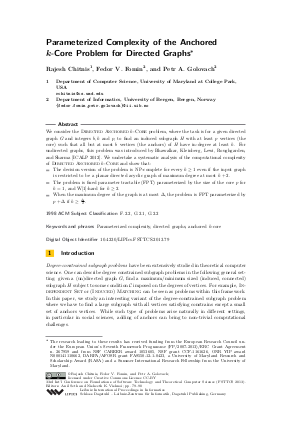Document# Parameterized Complexity of the Anchored k-Core Problem for Directed Graphs

### Authors Rajesh Chitnis, Fedor V. Fomin, Petr A. Golovach## File

LIPIcs.FSTTCS.2013.79.pdf
• Filesize: 461 kB
• 12 pages

## Cite As

Rajesh Chitnis, Fedor V. Fomin, and Petr A. Golovach. Parameterized Complexity of the Anchored k-Core Problem for Directed Graphs. In IARCS Annual Conference on Foundations of Software Technology and Theoretical Computer Science (FSTTCS 2013). Leibniz International Proceedings in Informatics (LIPIcs), Volume 24, pp. 79-90, Schloss Dagstuhl - Leibniz-Zentrum für Informatik (2013)
https://doi.org/10.4230/LIPIcs.FSTTCS.2013.79

## Abstract

We consider the Directed Anchored k-Core problem, where the task is for a given directed graph G and integers b, k and p, to find an induced subgraph H with at least p vertices (the core) such that all but at most b vertices (the anchors) of H have in-degree at least k. For undirected graphs, this problem was introduced by Bhawalkar, Kleinberg, Lewi, Roughgarden, and Sharma [ICALP 2012]. We undertake a systematic analysis of the computational complexity of Directed Anchored k-Core and show that: - The decision version of the problem is NP-complete for every k>=1 even if the input graph is restricted to be a planar directed acyclic graph of maximum degree at most k+2. - The problem is fixed parameter tractable (FPT) parameterized by the size of the core p for k=1, and W-hard for k>=2. - When the maximum degree of the graph is at most Delta, the problem is FPT parameterized by p+Delta if k>=Delta/2.
##### Keywords
• Parameterized complexity
• directed graphs
• anchored \$k\$-core

## Metrics

• Access Statistics
• Total Accesses (updated on a weekly basis)
0